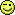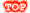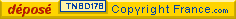Connectez-vous !Cliquez ici pour vous connecterNouveau compteDes millions de comptes créés.100% gratuit ![Avantages]Comme des milliers de personnes, recevez gratuitement chaque semaine une leçon d'anglais !Choisir RadioBBC (UK)VOA (US) - Accueil - Aide/Contact - Accès rapides- Lire cet extrait- Livre d'or- Nouveautés- Plan du site - Presse- Recommander- Signaler un bug - Traduire cet extrait- Webmasters- Lien sur votre site > Nos sites : -Jeux gratuits -Nos autres sites << Retour au forum || Aller tout en basCLIQUEZ ICI POUR REPONDRE A CE SUJET[Math] Trigonometry puzzleMessage de TravisKidd posté le 26-02-2007 à 08:42:47 (S | E | F | I) Here's a little trigonometry puzzle you might like: I. Using a calculator, calculate sin 7°, sin 53°, and sin 67°. Compare these values. What relation does there appear to be among them? II. Do the same thing for sin 23°, sin 37°, and sin 83°. III. Based on your discoveries in I. and II., hypothesize a trigonometric identity. IV. Prove this identity using the following well-known fact and identities: cos 60° = 1/2 sin(-x) = -(sin x) cos(-x) = cos x sin(x+y) = (sin x)(cos y) + (cos x)(sin y) V. Give a specific example of this identity using three specific values. (Two such examples are given in I. and II.) Show using a calculator that this identity applies to your three values. Good luck! I will post the correction here (in this thread) in approximately one week. ------------------- Modifié par bridg le 17-03-2007 15:56 transfert en maths Réponse: [Math] Trigonometry puzzle de magstmarc, postée le 27-02-2007 à 08:56:31 (S | E) Hi Travis, I. sin 7° is about 0,12 sin 53° is about 0,80 sin 67° is about 0,92 We can conjecture (does that word exist ?) that sin 67° = sin 53° + sin 7° II. sin 23° is about 0,39 sin 37° is about 0,60 sin 83° is about 0,99 We can hypothetise that sin 83° = sin 37° + sin 23° III. Conjecture : for every real number x, in degrees, sin(60° + x)= sin(60° - x)+ sin x IV. x being a real number, in degrees, sin(60°+x)= sin60°cosx + cos60°sinx and sin(60°-x)= sin[60° + (-x)] = sin60°cos(-x) + cos60°sin(-x) = sin60°cosx - cos60°sinx If we substract these two identities we get sin(60°+x) - sin(60°-x) = 2cos60°sinx = 2.(1/2).sin x = sin x that is, sin(60°+x) = sin(60°-x) + sin x V. So if x = 5°, sin 65° = sin 55° + sin 5° Calculator : sin 5° is about 0,09 sin 55° is about 0,82 sin 65° is about 0,91 Nice exercise TraviskiddCLIQUEZ ICI POUR REPONDRE A CE SUJET

>INDISPENSABLES : TESTEZ VOTRE NIVEAU | GUIDE DE TRAVAIL | NOS MEILLEURES FICHES | Les fiches les plus populaires | Une leçon par email par semaine | Exercices | Aide/Contact

> INSEREZ UN PEU D'ANGLAIS DANS VOTRE VIE QUOTIDIENNE ! Rejoignez-nous gratuitement sur les réseaux :
| Cours, leçons et exercices d'anglais 100% gratuits, hors abonnement internet auprès d'un fournisseur d'accès.| Livre d'or | Partager sur les réseaux